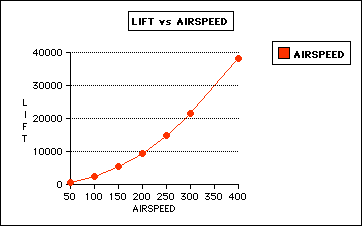+ Text Only Site
+ Non-Flash Version
+ Contact Glenn##Velocity Effects Answers1. Go to the Foilsim Software Program and set initial conditions as follows: Airspeed(velocity) = 50 km/h, Altitude = 5000 m, Angle = 1 deg , Thickness = 12.5, Camber = 12.5, Area = 5 sq. m.

What is the Lift? 595 Newtons

2. Change the velocity(airspeed) as shown in the following table and record the associated lift for each velocity to complete the table.
Velocity(km/h) - Airspeed
Lift(Newtons)
50
595
100
2,378
150
5,351
200
9,513
250
14,860
300
21,400
400
38,050
TABLE 1
3. Construct a graph of Velocity's effect on Lift with an Independent Variable(X) of Velocity and a Dependent Variable(Y) of Lift. Label each axis correctly to include units. Print out graph from Foilsim Program...4. What is the shape of the curve formed by this relationship? Parabolic Shape

5. Write the equation for this relationship! y = cx2__Where y = lift, and x = airspeed_and c = a constant

6. What is the value of the constant in this equation for this set of conditions?

C = .23784 Newtons/kph2

7. Interpolate the lift for a velocity of 350 kph. What is the lift? 29,130 Newtons

8. Extrapolate the lift for a velocity of 500 kph. What is the lift? 59,460 Newtons

9. Based on the relationship between lift and velocity, how would you increase the lift on a wing? Increase the Velocity

10. Set the initial conditions provided in Problem 1 above and assume: g = 9.8 m./sec.2 at this elevation; total weight of airplane, cargo, and fuel = 5000 kg.; weight of airplane = 3000 kg. ; weight of cargo and fuel = 2000 kg. Use the equation for lift that you generated in Problem 5 to answer these questions:
1. What is the lift in Newtons required to maintain altitude in these conditions?

49,000 Newtons

2. What is the velocity that is required to maintain this lift?

454 kph

11. One hour later all cargo is parachuted off the airplane and 200 kg. of fuel remains.
1. What is the lift in Newtons now required to maintain altitude?

31,360 Newtons

2. What is the velocity that is now required to generate the lift?

363 kph

Related Pages:
Standards
Activity
Worksheet
Lesson Index
Aerodynamics Index+ Inspector General Hotline + Equal Employment Opportunity Data Posted Pursuant to the No Fear Act + Budgets, Strategic Plans and Accountability Reports + Freedom of Information Act + The President's Management Agenda + NASA Privacy Statement, Disclaimer, and Accessibility CertificationEditor: Tom Benson NASA Official: Tom Benson Last Updated: Thu, Jun 12 04:46:42 PM EDT 2014 + Contact Glenn